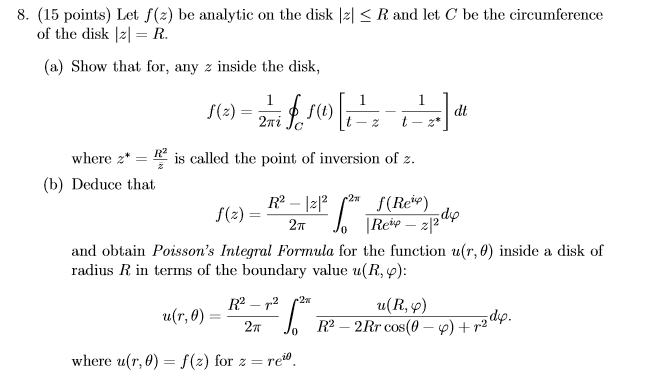# Integration (related to contour integration)

equalPAs the tutorial has not covered this topic yet, I'm quite confused on this question. (there is another question about this topic too, but I would like to try that question after knowing how to do this question)
Q.8 (a) (I'm not sure whether I will know how to do (b) after having (a) done, I just ask (a) first)
$C: t=Re^{i\theta}\\ dt=iRe^{i\theta}d\theta\\ R.H.S.=\frac{1}{2\pi i}\oint_{C}f(t)[\frac{1}{t-z}-\frac{1}{t-z^*}]dt\\ =\frac{1}{2\pi i}\oint_{C}\frac{f(t)}{t-z}dt-\frac{1}{2\pi i}\oint_{C}\frac{f(t)}{t-z^*}dt\\ =\frac{1}{2\pi i}\int_{0}^{2\pi}\frac{f(Re^{i\theta})}{Re^{i\theta}-z}iRe^{i\theta}d\theta-\frac{1}{2\pi i}\int_{0}^{2\pi}\frac{f(Re^{i\theta})}{Re^{i\theta}-z^*}iRe^{i\theta}d\theta\\ =\frac{R}{2\pi}\int_{0}^{2\pi}\frac{f(Re^{i\theta})}{Re^{i\theta}-z}e^{i\theta}d\theta-\frac{R}{2\pi}\int_{0}^{2\pi}\frac{f(Re^{i\theta})}{Re^{i\theta}-z^*}e^{i\theta}d\theta$

I'm confused that whether I should do it like this.
When I go to this step, I do not know what should I do to integrate it.
I have tried integration by part but I then got a more complicated one...
Can anyone help me? Thank you.